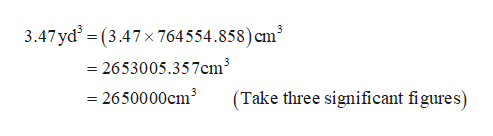How many cubic centimeters are there in 3.47yd^3Express the volume in cubic centimeters to three significant figures.

Question

How many cubic centimeters are there in 3.47yd^3
Express the volume in cubic centimeters to three significant figures.

Step 1

It is known that 1 yard is equal to 91.44Cm.

Then 1yd...help_outlineImage Transcriptionclose3.47 yd2 = (3.47x 764554.858) cm' =2653005.357cm3 = 2650000cm3 (Take three significant figures) fullscreen

Want to see the full answer?

See Solution

Want to see this answer and more?

Our solutions are written by experts, many with advanced degrees, and available 24/7

See Solution
Tagged in

Math